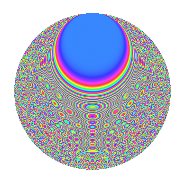# Properties

 Label 2541.2.nLevel 2541 Weight 2 Character orbit n Rep. character $$\chi_{2541}(122,\cdot)$$ Character field $$\Q(\zeta_{6})$$ Dimension 546 Sturm bound 704

# Related objects

## Defining parameters

 Level: $$N$$ = $$2541 = 3 \cdot 7 \cdot 11^{2}$$ Weight: $$k$$ = $$2$$ Character orbit: $$[\chi]$$ = 2541.n (of order $$6$$ and degree $$2$$) Character conductor: $$\operatorname{cond}(\chi)$$ = $$21$$ Character field: $$\Q(\zeta_{6})$$ Sturm bound: $$704$$

## Dimensions

The following table gives the dimensions of various subspaces of $$M_{2}(2541, [\chi])$$.

Total New Old
Modular forms 752 618 134
Cusp forms 656 546 110
Eisenstein series 96 72 24

## Trace form

 $$546q + 3q^{3} + 258q^{4} + 5q^{7} + q^{9} + O(q^{10})$$ $$546q + 3q^{3} + 258q^{4} + 5q^{7} + q^{9} - 6q^{12} + 4q^{15} - 216q^{16} + 18q^{18} + 3q^{19} + 8q^{21} - 36q^{24} - 203q^{25} + 32q^{28} + 20q^{30} + 39q^{31} - 4q^{36} + 3q^{37} - 23q^{39} - 60q^{40} + 56q^{42} - 26q^{43} + 24q^{45} - 4q^{46} - q^{49} + 16q^{51} - 6q^{52} - 66q^{54} + 26q^{57} + 24q^{58} - 60q^{60} + 24q^{61} + 9q^{63} - 312q^{64} - 11q^{67} + 84q^{70} - 36q^{72} + 33q^{73} - 57q^{75} - 188q^{78} - 27q^{79} - 51q^{81} + 60q^{82} - 2q^{84} - 8q^{85} - 18q^{87} + 9q^{91} + 17q^{93} - 48q^{94} + 18q^{96} + O(q^{100})$$

## Decomposition of $$S_{2}^{\mathrm{new}}(2541, [\chi])$$ into newform subspaces

The newforms in this space have not yet been added to the LMFDB.

## Decomposition of $$S_{2}^{\mathrm{old}}(2541, [\chi])$$ into lower level spaces

$$S_{2}^{\mathrm{old}}(2541, [\chi]) \cong$$ $$S_{2}^{\mathrm{new}}(21, [\chi])$$$$^{\oplus 3}$$$$\oplus$$$$S_{2}^{\mathrm{new}}(231, [\chi])$$$$^{\oplus 2}$$

## Hecke Characteristic Polynomials

There are no characteristic polynomials of Hecke operators in the database